|
|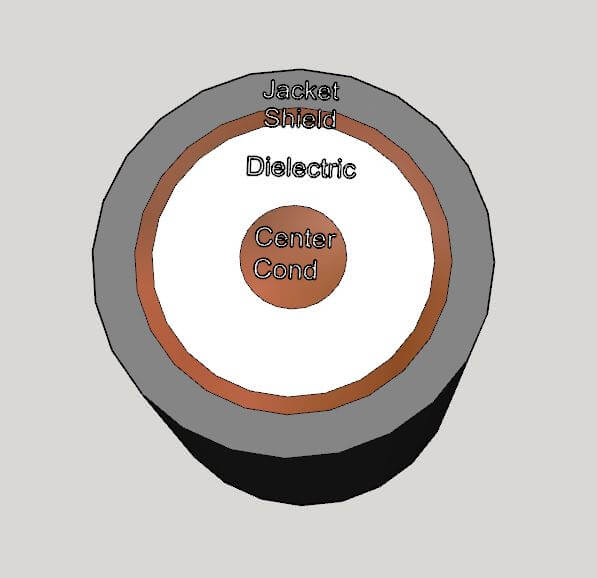### What is the Characteristic Impedance of a Coaxial Cable?

March 22, 2023

A coaxial cable is an RF transmission line which consists of a center conductor surrounded by a dielectric medium, a coaxial shield, and often an insulating plastic jacket.Figure 1 – Coaxial Cable

The inner conductor of the coaxial cable possesses an intrinsic inductance per unit length L’ just like the wire in free space. By virtue of the coaxial shield and the dielectric material, it also has a capacitance per unit length C’. The inductance and capacitance are distributed along the length of the cable, as shown in Figure 4 below.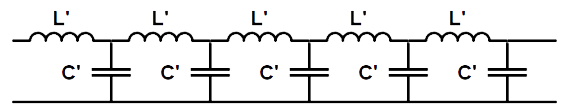Figure 2 – Inductance and Capacitance per Unit Length of a Coaxial Cable

If the inductance and capacitance per unit length are known, then the characteristic impedance of the cable is given by: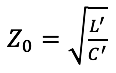Low-loss dielectric materials such as PTFE are common. PTFE has a relative dielectric constant of 2.02 to 2.1, but this is commonly processed to create a PTFE foam with a significant amount of air, resulting in lower loss and a lower dielectric constant.

RF travels through the coaxial cable at the guide velocity vg, slower than the speed of light, c: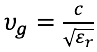The relative velocity of propagation is expressed as the percentage of c or: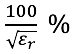This number is approximately 65% for solid PTFE cables, and as high as 90% for low loss foamed PTFE. An air-line coaxial cable with only air as a dielectric would have a relative velocity of 100%.

The characteristic impedance of a coaxial transmission line may be calculated from:Where Douter is the diameter of the outer shield to its inner surface, Dcenter is the diameter of the center conductor, and ∈r is the relative dielectric constant of the medium in between the two.

See this and more important radio frequency charts and formulas in this comprehensive application note